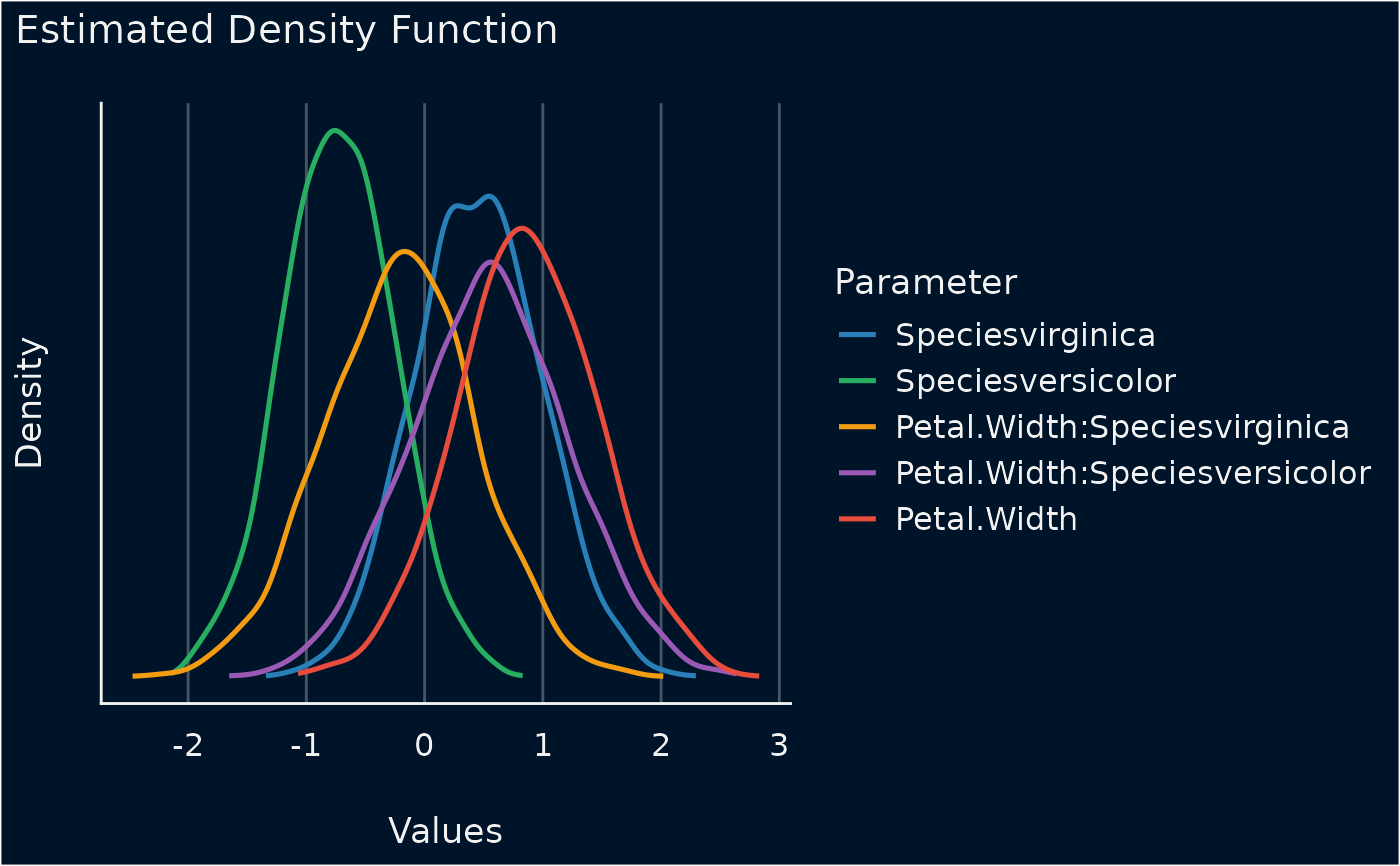The plot() method for the bayestestR::estimate_density() function.

## Usage

# S3 method for see_estimate_density
plot(
x,
stack = TRUE,
show_intercept = FALSE,
n_columns = 1,
priors = FALSE,
priors_alpha = 0.4,
posteriors_alpha = 0.7,
size_line = 0.9,
size_point = 2,
centrality = "median",
ci = 0.95,
...
)

## Arguments

x

An object.

stack

Logical. If TRUE, densities are plotted as stacked lines. Else, densities are plotted for each parameter among each other.

show_intercept

Logical, if TRUE, the intercept-parameter is included in the plot. By default, it is hidden because in many cases the intercept-parameter has a posterior distribution on a very different location, so density curves of posterior distributions for other parameters are hardly visible.

n_columns

For models with multiple components (like fixed and random, count and zero-inflated), defines the number of columns for the panel-layout. If NULL, a single, integrated plot is shown.

priors

Logical. If TRUE, prior distributions are simulated (using bayestestR::simulate_prior()) and added to the plot.

priors_alpha

Numeric value specifying alpha for the prior distributions.

posteriors_alpha

Numeric value specifying alpha for the posterior distributions.

size_line

Numeric value specifying size of line geoms.

size_point

Numeric specifying size of point-geoms.

centrality

Character specifying the point-estimate (centrality index) to compute. Can be "median", "mean" or "MAP".

ci

Numeric value of probability of the CI (between 0 and 1) to be estimated. Default to .95.

...

Arguments passed to or from other methods.

## Value

A ggplot2-object.

## Examples

# \donttest{
if (require("bayestestR") && require("rstanarm")) {
set.seed(123)
m <<- stan_glm(Sepal.Length ~ Petal.Width * Species, data = iris, refresh = 0)
result <- estimate_density(m)
plot(result)
}# }